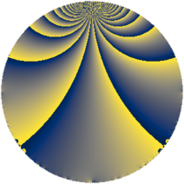# Properties

 Label 77.2.nLevel $77$ Weight $2$ Character orbit 77.n Rep. character $\chi_{77}(17,\cdot)$ Character field $\Q(\zeta_{30})$ Dimension $48$ Newform subspaces $1$ Sturm bound $16$ Trace bound $0$

# Related objects

## Defining parameters

 Level: $$N$$ $$=$$ $$77 = 7 \cdot 11$$ Weight: $$k$$ $$=$$ $$2$$ Character orbit: $$[\chi]$$ $$=$$ 77.n (of order $$30$$ and degree $$8$$) Character conductor: $$\operatorname{cond}(\chi)$$ $$=$$ $$77$$ Character field: $$\Q(\zeta_{30})$$ Newform subspaces: $$1$$ Sturm bound: $$16$$ Trace bound: $$0$$

## Dimensions

The following table gives the dimensions of various subspaces of $$M_{2}(77, [\chi])$$.

Total New Old
Modular forms 80 80 0
Cusp forms 48 48 0
Eisenstein series 32 32 0

## Trace form

 $$48q - 5q^{2} - 9q^{3} - 9q^{4} - 15q^{5} - 5q^{7} - 11q^{9} + O(q^{10})$$ $$48q - 5q^{2} - 9q^{3} - 9q^{4} - 15q^{5} - 5q^{7} - 11q^{9} - q^{11} - 12q^{12} - 8q^{14} - 27q^{16} + 15q^{17} + 20q^{18} - 15q^{19} - 76q^{22} + 10q^{23} + 75q^{24} + q^{25} + 27q^{26} - 40q^{28} - 40q^{29} + 25q^{30} + 9q^{31} + 42q^{33} + 5q^{35} - 38q^{36} - q^{37} + 33q^{38} - 45q^{39} + 75q^{40} + 64q^{42} + 30q^{44} - 84q^{45} - 20q^{46} + 3q^{47} + 59q^{49} + 30q^{50} + 55q^{51} - 15q^{52} - 3q^{53} - 8q^{56} + 60q^{57} + 46q^{58} - 3q^{59} - 15q^{60} - 30q^{61} - 40q^{63} + 12q^{64} - 93q^{66} + 44q^{67} - 75q^{68} - 27q^{70} + 20q^{71} - 60q^{72} - 60q^{73} + 45q^{74} - 57q^{75} + 92q^{78} - 70q^{79} - 75q^{80} - 29q^{81} - 129q^{82} - 125q^{84} + 10q^{85} - 62q^{86} + 19q^{88} + 6q^{89} - 12q^{91} + 30q^{92} - 92q^{93} + 105q^{94} + 30q^{95} + 75q^{96} - 12q^{99} + O(q^{100})$$

## Decomposition of $$S_{2}^{\mathrm{new}}(77, [\chi])$$ into newform subspaces

Label Dim. $$A$$ Field CM Traces $q$-expansion
$$a_2$$ $$a_3$$ $$a_5$$ $$a_7$$
77.2.n.a $$48$$ $$0.615$$ None $$-5$$ $$-9$$ $$-15$$ $$-5$$